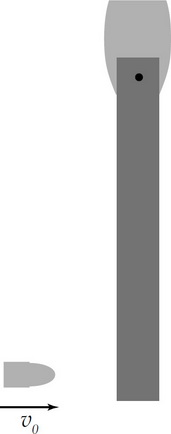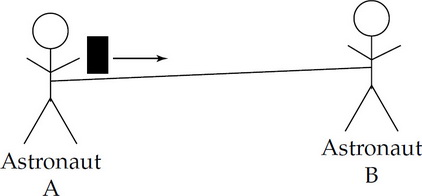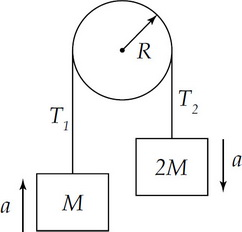# AP Physics C: Mechanics Practice Test 6

### Test Information11 questions15 minutes

1. A ball with a radius of 0.2 m rolls without slipping on a level surface. The center of mass of the ball moves at a constant velocity, moving a distance of 30 meters in 10 seconds. The angular speed of the ball about its point of contact on the surface is

2.A bullet is moving with a velocity v0 when it collides with and becomes embedded in a wooden bar that is hinged at one end, as shown above. Consider the bullet and the wooden bar to be the system. For this scenario, which of the following is true?

3. A spring mass system is vibrating along a frictionless, horizontal floor. The spring constant is 8 N/m, the amplitude is 5 cm and the period is 4 seconds.

In kg, the mass of the system is

4. A spring mass system is vibrating along a frictionless, horizontal floor. The spring constant is 8 N/m, the amplitude is 5 cm and the period is 4 seconds.

Which of the following equations could represent the position of the mass from equilibrium x as a function of time t, where x is in meters and t is in seconds.

5. Two blocks of masses M and 3M are connected by a light string. The string passes over a frictionless pulley of negligible mass so that the blocks hang vertically. The blocks are then released from rest. What is the acceleration of the mass M?

6. For a particular nonlinear spring, the relationship between the magnitude of the applied force F and the stretch of the spring x is given by the equation F = kx1.5. How much energy is stored in the spring when is it stretched a distance x1?

7. Two ice skaters are moving on frictionless ice and are about to collide. The 50-kg skater is moving directly west at 4 m/s. The 75-kg skater is moving directly north at 2 m/s. After the collision they stick together.

What is the magnitude of the momentum of the two-skater system after the collision?

8. Two ice skaters are moving on frictionless ice and are about to collide. The 50-kg skater is moving directly west at 4 m/s. The 75-kg skater is moving directly north at 2 m/s. After the collision they stick together.

For this scenario, which of the following is true?

9. The position of an object is given by the equations x = 2.0t3 + 4.0t + 6.25, where x is in meters and t is in seconds. What is the acceleration of the object at t = 1.50 s?

10.Two astronauts are at rest out in space and connected to a rope that is taut, as shown above. Astronaut A throws a heavy oxygen container towards Astronaut B, who then catches the container. Which of the following describes the motion of Astronaut B?

Immediately After the Throw &nbps;&nbps;&nbps; After the Catch

11.Two blocks of masses M and 2M are connected by a light string. The string passes over a pulley, as shown above. The pulley has a radius R and moment of inertia I about its center. t1 and t2 are the tensions in the string on each side of the pulley and a is the acceleration of the masses. Which of the following equations best describes the pulley's rotational motion during the time the blocks accelerate?